# US8384588B2 - Beam stabilization for wideband phase comparison monopulse angle estimation with electronically steered antennas - Google Patents

Beam stabilization for wideband phase comparison monopulse angle estimation with electronically steered antennas Download PDF

## Info

Publication number
US8384588B2
US8384588B2 US12/925,668 US92566810A US8384588B2 US 8384588 B2 US8384588 B2 US 8384588B2 US 92566810 A US92566810 A US 92566810A US 8384588 B2 US8384588 B2 US 8384588B2
Authority
US
United States
Prior art keywords
spatial frequency
monopulse
frequency data
wideband
signal
Prior art date
Legal status (The legal status is an assumption and is not a legal conclusion. Google has not performed a legal analysis and makes no representation as to the accuracy of the status listed.)
Active, expires
Application number
US12/925,668
Other versions
US20120098694A1 (en
Inventor
Charles T. Hansen
Current Assignee (The listed assignees may be inaccurate. Google has not performed a legal analysis and makes no representation or warranty as to the accuracy of the list.)
Raytheon Co
Original Assignee
Raytheon Co
Priority date (The priority date is an assumption and is not a legal conclusion. Google has not performed a legal analysis and makes no representation as to the accuracy of the date listed.)
Filing date
Publication date
Application filed by Raytheon Co filed Critical Raytheon Co
Priority to US12/925,668 priority Critical patent/US8384588B2/en
Assigned to RAYTHEON COMPANY reassignment RAYTHEON COMPANY ASSIGNMENT OF ASSIGNORS INTEREST (SEE DOCUMENT FOR DETAILS). Assignors: HANSEN, CHARLES T.
Publication of US20120098694A1 publication Critical patent/US20120098694A1/en
Application granted granted Critical
Publication of US8384588B2 publication Critical patent/US8384588B2/en
Active legal-status Critical Current

• 230000000087 stabilizing Effects 0.000 claims abstract description 8
• 238000010606 normalization Methods 0.000 claims description 6
• 239000003381 stabilizers Substances 0.000 claims description 6
• 230000001131 transforming Effects 0.000 claims description 3
• 238000010586 diagrams Methods 0.000 description 8
• 230000001419 dependent Effects 0.000 description 7
• 239000000203 mixtures Substances 0.000 description 6
• 238000004364 calculation methods Methods 0.000 description 4
• 235000020127 ayran Nutrition 0.000 description 3
• 230000000875 corresponding Effects 0.000 description 3
• 230000014509 gene expression Effects 0.000 description 3
• 238000000034 methods Methods 0.000 description 3
• 239000000969 carriers Substances 0.000 description 2
• 239000011159 matrix materials Substances 0.000 description 2
• 238000001228 spectrum Methods 0.000 description 2
• 239000003570 air Substances 0.000 description 1
• 230000005540 biological transmission Effects 0.000 description 1
• 230000004048 modification Effects 0.000 description 1
• 238000006011 modification reactions Methods 0.000 description 1

## Images

••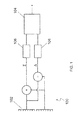•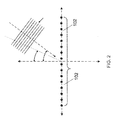••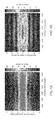•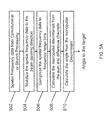•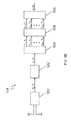•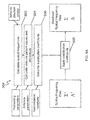•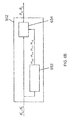•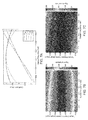•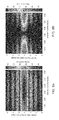•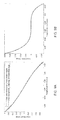## Classifications

• GPHYSICS
• G01MEASURING; TESTING
• G01S13/00Systems using the reflection or reradiation of radio waves, e.g. radar systems; Analogous systems using reflection or reradiation of waves whose nature or wavelength is irrelevant or unspecified
• G01S13/02Systems using reflection of radio waves, e.g. primary radar systems; Analogous systems
• G01S13/06Systems determining position data of a target
• G01S13/42Simultaneous measurement of distance and other co-ordinates
• G01S13/44Monopulse radar, i.e. simultaneous lobing
• G01S13/4454Monopulse radar, i.e. simultaneous lobing phase comparisons monopulse, i.e. comparing the echo signals received by an interferometric antenna arrangement

## Abstract

A method for estimating a target angle of a wideband signal received on an electronically steered antenna array includes: generating spatial frequency data from the received wideband signal; stabilizing the spatial frequency data to a beam steering direction; compressing the stabilized spatial frequency data to a plurality of frequency range bins; calculating a monopulse discriminant from the stabilized spatial frequency data; and calculating the target angle using the monopulse discriminant.

## Description

STATEMENT REGARDING FEDERALLY SPONSORED RESEARCH OR DEVELOPMENT

This invention disclosure is related to Government contract F33615-02-D-1116 awarded by the Department of the Air Force. The Government has certain rights in this invention.

BACKGROUND

Phase comparison monopulse angle estimation is a well known technique for measuring angle of arrival of a signal in a radar or other radio frequency electromagnetic sensor. To use this technique, the receiving antenna is typically divided into two halves. The angle of arrival is a function of the ratio of the difference signal (created by subtracting the signal from the two halves) divided by the sum signal (created by adding the two halves). This value is known as the monopulse discriminant.

Generally, the algorithm that performs the angle estimation using the monopulse discriminant relies on the assumption that the value of the monopulse discriminant does not substantially vary as a function of the frequencies that make up the signal. However, this assumption does not hold true for wideband or spread spectrum waveforms or signals when they are measured by an electronically scanned phased array antenna.

SUMMARY

Embodiments of the present invention are directed to systems and methods for computing a monopulse discriminant that is substantially frequency invariant even for phase steered antennas when wideband waveforms are used. This frequency invariant monopulse discriminant can be used to compute the angle of arrival of wideband signals.

According to one embodiment of the present invention, a method for estimating a target angle of a wideband signal received on an electronically steered antenna array includes: generating spatial frequency data from the received wideband signal; stabilizing the spatial frequency data to a beam steering direction; compressing the stabilized spatial frequency data to a plurality of frequency range bins; calculating a monopulse discriminant from the stabilized spatial frequency data; and calculating the target angle using the monopulse discriminant.

According to another embodiment of the present invention, a system for stabilizing wideband spatial frequency data for estimating a target angle of a wideband signal on an electronically steered antenna array includes: a spatial frequency processor for transforming the wideband signal to spatial frequency data; a signal stabilizer configured to stabilize the spatial frequency data; a frequency to range transformer configured to compress the stabilized spatial frequency data into a plurality of frequency range bins; a monopulse discriminant calculator for calculating a monopulse discriminant from the compressed stabilized spatial frequency data; and a target angle calculator configured to calculate the target angle using the monopulse discriminant.

According to another embodiment of the present invention, a wideband monopulse radar system includes: an antenna array comprising a plurality of antennas for receiving a wideband monopulse signal; a signal processor for estimating a target angle of the wideband monopulse signal, the signal processor being configured to: transform the wideband monopulse signal to wideband spatial frequency data, stabilize spatial frequency data of the wideband monopulse signal to a beam steering direction; compress the stabilized spatial frequency data to a plurality of frequency range bins; calculate a monopulse discriminant from the compressed stabilized spatial frequency data; and calculate the target angle using the monopulse discriminant.

BRIEF DESCRIPTION OF THE DRAWINGS

The patent or application file contains at least one drawing executed in color. Copies of this patent or patent application publication with color drawing(s) will be provided by the Office upon request and payment of the necessary fee.

FIG. 1 is a block diagram of a radar system according to one embodiment of the present invention.

FIG. 2 is a diagram illustrating the arrival of a wideband signal on an antenna array according to one embodiment of the present invention.

FIG. 3A is a diagram illustrating a calculated target angle for a lowest frequency and a highest frequency of an unstabilized wideband signal according to a set of example conditions.

FIGS. 3B and 3C are graphs illustrating signal amplitude of an unstabilized wideband signal with respect to signal frequency and angle for a sum channel and a difference channel, respectively, according to a set of example conditions.

FIGS. 4A and 4B are graphs illustrating impulse responses of an unstabilized wideband signal for a sum channel and a difference channel, respectively according to a set of example conditions.

FIG. 5A is a flowchart illustrating a method of determining an angle to a target from unstabilized spatial frequency data according to one embodiment of the present invention.

FIG. 5B is a block diagram illustrating a signal processor for determining a target angle from spatial frequency data according to one embodiment of the present invention.

FIG. 6A is a flowchart illustrating a method of stabilizing spatial frequency data according to one embodiment of the present invention.

FIG. 6B is a block diagram illustrating a signal stabilizer according to one embodiment of the present invention.

FIG. 7A is a graph showing the values of stabilization coefficients calculated according to one embodiment of the present invention for a set of example conditions.

FIGS. 7B and 7C are graphs illustrating signal amplitude of a stabilized wideband signal with respect to signal frequency and angle for a sum channel and a difference channel, respectively, according to one embodiment of the present invention for a set of example conditions.

FIGS. 8A and 8B are graphs depicting the impulse response for the sum and difference channels of the example conditions, respectively, according to one embodiment of the present invention for a set of example conditions.

FIG. 9A is a graph comparing an ideal discriminant for a narrow band signal with a discriminant derived from the stabilized impulse response over a direction.

FIG. 9B is a graph of the difference between the ideal discriminant for the narrow band signal and the discriminant derived from the stabilized impulse response.

DETAILED DESCRIPTION

According to one embodiment of the present invention, phase comparison monopulse angle estimation may be used in a radar system 100 such as one shown in block diagram form in FIG. 1. An antenna 102 receives a signal which is split into sum and difference channels and supplied to analog-digital converters 106. A signal processor 104 may be used to analyze the received signals. The system 100 may also include filters, oscillators, and other analog and digital signal processing components as are well known in the art.

Angle estimation of radar returns is often done with an antenna having two channels: a sum channel and a difference channel. The angle of arrival of a received signal (or, equivalently, due to antenna reciprocity theorem, the transmission angle of an outgoing signal) is computed using the sum and difference channels, but the relationship between the angle of arrival and the relative amplitude of the received signal depends on the frequency of the signal when the antenna is steered using adjustment of phase between the elements. As a result, these angle estimation techniques are generally used with narrow band signals rather than wide band signals because there is not a well defined mapping between angle and relative amplitude of the signal for wide band signal. That is, the wide band signals have a range of frequencies, making it difficult to calculate a single angle of arrival for all of the frequencies. Therefore, a substantially frequency independent method of determining the angle of arrival would be useful.

To illustrate the problem, consider a simplified antenna model in which the antenna consists only of two omnidirectional elements spaced along a length L in the x direction. Suppose that a sinusoidal signal s having a frequency ω is incident on the antenna with angle of incidence θ. The signals (s0 and s1) on the two halves of the antenna will be:

$s 0 = exp ⁡ [ - ⅈω ⁡ ( t + L 2 ⁢ c ⁢ sin ⁢ ⁢ θ ) ]$ $s 1 = exp ⁡ [ - ⅈω ⁡ ( t - L 2 ⁢ c ⁢ sin ⁢ ⁢ θ ) ]$

Therefore, the sum (sΣ) of the two signals is:

$s Σ = exp ⁡ [ - ⅈω ⁢ ⁢ t ] ⁢ ( exp ⁡ [ - ⅈ ⁢ ω ⁢ ⁢ L 2 ⁢ c ⁢ sin ⁢ ⁢ θ ] + exp ⁡ [ ⅈ ⁢ ω ⁢ ⁢ L 2 ⁢ c ⁢ sin ⁢ ⁢ θ ] )$ $s Σ = exp ⁡ [ - ⅈω ⁢ ⁢ t ] ⁢ ( 2 ⁢ ⁢ cos ⁡ [ ω ⁢ ⁢ L 2 ⁢ c ⁢ sin ⁢ ⁢ θ ] )$

Similarly, the difference (sΔ) of the two signals is:

$s Δ = exp ⁡ [ - ⅈω ⁢ ⁢ t ] ⁢ ( 2 ⁢ ⅈ ⁢ ⁢ sin ⁡ [ ω ⁢ ⁢ L 2 ⁢ c ⁢ sin ⁢ ⁢ θ ] )$

Therefore, the monopulse discriminant (sΔ/sΣ) is:

$s Δ s Σ - ⅈ ⁢ ⁢ tan ⁡ [ ω ⁢ ⁢ L 2 ⁢ c ⁢ sin ⁢ ⁢ θ ]$

Note that this expression can be used to determine the angle of arrival θ from the monopulse discriminant (sΔ/sΣ) as long as

$ ω ⁢ ⁢ L 2 ⁢ c ⁢ sin ⁢ ⁢ θ  < π 2 .$
Note also that the relationship depends on the frequency of the signal (ω). If this condition is not satisfied, then the relationship between the monopulse discriminant and angle of arrival admits multiple solutions, which means that the angle of arrival may be ambiguous.

Electronic steering can be used to determine the angle of arrival for signals that do not satisfy the condition

$ ω ⁢ ⁢ L 2 ⁢ c ⁢ sin ⁢ ⁢ θ  < π 2 .$
This is done by applying a phase (e.g.,

$( e . g . , + ⅈ ⁢ ⁢ θ s 2 ⁢ ⁢ and ⁢ - θ s 2 )$
to each element. That is:

$s 0 = exp ⁡ [ - ⅈω ⁡ ( t + L 2 ⁢ c ⁢ sin ⁢ ⁢ θ ) + ⅈ ⁢ ⁢ θ s 2 ]$ $s 1 = exp ⁡ [ - ⅈω ⁡ ( t - L 2 ⁢ c ⁢ sin ⁢ ⁢ θ ) - ⅈ ⁢ ⁢ θ s 2 ]$

When these channels are combined, they form a monopulse discriminant that looks like:

$s Δ s Σ = ⅈ ⁢ ⁢ tan ⁡ [ ω ⁢ ⁢ L 2 ⁢ c ⁢ sin ⁢ ⁢ θ - θ s 2 ]$

Similarly, the electronically steered array can be used to determine the angle of arrival for signals satisfying the condition

$ ω ⁢ ⁢ L 2 ⁢ c ⁢ sin ⁢ ⁢ θ - θ s 2  < π 2$
and therefore θ satisfies the condition:

$ ω ⁢ ⁢ L 2 ⁢ c ⁢ sin ⁢ ⁢ θ - θ s 2  < π 2 ⁢ - π 2 < ω ⁢ ⁢ L 2 ⁢ c ⁢ sin ⁢ ⁢ θ - θ s 2 < π 2$ $θ s 2 - π 2 < ω ⁢ ⁢ L 2 ⁢ c ⁢ sin ⁢ ⁢ θ < θ s 2 + π 2$ $c ω ⁢ ⁢ L ⁢ ( θ s - π ) < sin ⁢ ⁢ θ < c ω ⁢ ⁢ L ⁢ ( θ s + π )$ $arcsin ⁡ [ c ω ⁢ ⁢ L ⁢ ( θ s - π ) ] < θ < arcsin ⁡ [ c ω ⁢ ⁢ L ⁢ ( θ s + π ) ]$

Therefore, the angle of arrival is centered on:

$θ look = arcsin ⁡ [ θ s ⁢ c ω ⁢ ⁢ L ]$

In other words, the nominal look direction of the array (also referred to as a “steering angle” or “steering direction”) is equal to θlook, which depends on the frequency ω. Using the above described method to determine the angle of arrival is simple for single frequency or narrow band signals because there is little or no variation in frequency ω such that there is little or no variation in the computed direction θlook.

However, using the above described method to compute the angle of arrival of a wide band signal can be difficult because the above-developed monopulse discriminant is frequency dependent and a wide band signal includes a wide range of frequencies.

Assume, for example, a wide band signal incident on an antenna array that is steered to 60 degrees away from boresight. Assume there are sixteen receiving elements in the array with unity spacing see FIG. 2. Assume also that the center frequency to be considered has a wavelength equal to precisely twice the array spacing. Therefore, the spatial frequency of the center of the band to be considered is equal to π radians.

For a signal at the center spatial frequency, the half power width of the beam in direction cosine space is approximately 1/16

$( 1 2 ⁢ λ L = 1 16 ≈ 3.58 ⁢ ° ,$
where L=16 is the length of the array and λ=2 as twice the array spacing) The maximum width of the beam at a given steering angle θlook is given by

$1 cos ⁢ ⁢ θ look ⁢ λ 2 ⁢ L ,$
which can be derived as follows:

$sin ⁢ ⁢ θ look - c ⁢ ⁢ π ω ⁢ ⁢ L < sin ⁢ ⁢ θ < sin ⁢ ⁢ θ look + c ⁢ ⁢ π ω ⁢ ⁢ L - c ⁢ ⁢ π ω ⁢ ⁢ L < sin ⁢ ⁢ θ - sin ⁢ ⁢ θ look < c ⁢ ⁢ π ω ⁢ ⁢ L - c ⁢ ⁢ π ω ⁢ ⁢ L < sin ⁡ ( θ look + δθ ) - sin ⁢ ⁢ θ look < c ⁢ ⁢ π ω ⁢ ⁢ L$ $sin ⁡ ( θ look + δθ ) ≈ sin ⁢ ⁢ θ look + cos ⁢ ⁢ θ look ⁢ δθ - c ⁢ ⁢ π ω ⁢ ⁢ L < cos ⁢ ⁢ θ look ⁢ δθ < c ⁢ ⁢ π ω ⁢ ⁢ L < c ⁢ ⁢ π ω ⁢ ⁢ L$ $⁢ δθ < 1 cos ⁢ ⁢ θ look ⁢ c ⁢ ⁢ π ω ⁢ ⁢ L$ $⁢ c ω = c 2 ⁢ π ⁢ ⁢ f = λ 2 ⁢ π$ $⁢ δθ < 1 cos ⁢ ⁢ θ look ⁢ λ 2 ⁢ L$

For example, at a steering angle of 60 degrees (i.e., direction cosine of about 0.86), this translates to δθ less than about 7.2 degrees, that is, the beam width is about 7.2 degrees.

The maximum deviation of the beam ∂θlook due to frequency can be computed as follows:

$sin ⁢ ⁢ θ look = θ s ⁢ c ω ⁢ ⁢ L$ $∂ sin ⁢ ⁢ θ look = ⁢ - θ s ⁢ c L ⁢ 1 ω 2 ⁢ ∂ ω = ⁢ - θ s ⁢ c L ⁢ 1 ω ⁢ ∂ ω ω = ⁢ - sin ⁢ ⁢ θ look ⁢ ∂ ω ω$ $∂ sin ⁢ ⁢ θ look = cos ⁢ ⁢ θ look ⁢ ∂ θ look$ $∂ θ look = - tan ⁢ ⁢ θ look ⁢ ∂ ω ω$

For example, if the bandwidth to be collected is equal to 20 percent of the center frequency

$( ∂ ω ω = 0.2 ) ,$
then, at a steering angle of 60 degrees (θlook=60°), the maximum deviation of the beam ∂θlook due to frequency variation will be about 19.8 degrees. Therefore, under these example conditions, the deviation of the beam across the frequency is comparable to or larger than the width of the beam (about 7.2 degrees to one side).

FIG. 3A illustrates a beam pattern of a low frequency of a waveform (red lobe 302) and a beam pattern of a high frequency of the waveform (blue lobe 304). This illustration assumes that phase steering is used and that the phase steering is defined to place the waveform center frequency at the desired direction. Since the beam pointing angle is dependent on frequency for a phase steered antenna, the higher frequencies will be directed closer to boresight and the lower frequencies will be directed away from boresight. FIGS. 3B and 3C are graphs illustrating beam power or amplitude (shown in color) of the sum and difference channels, respectively, as a function of spatial frequency along the horizontal axis and target direction (or angle) along the vertical axis. For a single frequency, the antenna patterns change in a consistent way as a function of angle. As can be seen from FIGS. 3B and 3C, for a given target direction, the beam power changes across the frequency spectrum (that is, the beam power is not substantially constant for a given target direction across the frequency range). In other words, the angle of arrival and the frequency are dependent on one another, thereby making it difficult to determine the target direction when using a wide band signal.

FIGS. 4A and 4B depict the impulse response of the sum and difference channels, respectively, over a range of direction cosines (or target angles). These impulse responses are formed by taking the Fourier transform of the spatial frequency signals in FIGS. 3B and 3C. Since the spatial frequency and angle of arrival are dependent on one another, it follows that the impulse response will also be dependent on the angle of arrival. This means that targets at different angles will have different impulse responses. If we imagine the case in which there are multiple targets separated in both range and angle, it is apparent that the sum and difference channels are quite different from one another and that it may be difficult, if not impossible to isolate a single target in both channels simultaneously, especially in the case of many competing signals.

For example, as can be seen in the impulse response of the sum channel in FIG. 4A, the shape of the impulse response signal varies over the direction cosine (or angle) along the x-axis. As a result, it may be difficult to map a particular received signal with a target direction. As another example, as can be seen in the impulse response of the difference channel in FIG. 4B, there is a low value (or hole) at a range of 0 and the steering angle (in this example, at about 0.866), but the shape of the impulse is not constant or similar along the range axis. To summarize, the angle-frequency relationship creates an angle dependent impulse response shape, so if the actual range to the target is unknown, or if there are multiple targets, there is no clear way to form a monopulse discriminant.

However, it is possible to combine the sum and difference channels in such a way that the impulse responses have the same shape for both the effective sum and difference channels. That is, it is possible to generate sum channels such that the impulse responses are substantially constant across the angles to the targets and to generate difference channels. In addition, this also means that it is possible for the monopulse discriminant to be used for accurate calculation of a target direction.

Embodiments of the present invention are directed toward methods and systems for accurately estimating the angle of arrival of a wide band signal. The methods and systems use signal processing to synthesize the sum and difference channels to provide a single mapping between angle and relative amplitude for all frequencies by creating a linear combination of the original channels and using combination coefficients that depend on frequency.

According to one embodiment of the present invention, conditions are imposed on the monopulse discriminant so that it is independent of frequency (for the sake of convenience, referred to hereafter as a “monopulse discriminant”). Those conditions are:

1. The monopulse discriminant is zero in the look direction for all frequencies.

2. The slope of the monopulse discriminant is frequency independent.

3. The value of the sum channel is normalized to the center frequency.

4. The value of the derivative of the difference channel is normalized to the center frequency.

1. The far field antenna patterns can be represented or approximated by a sum of discrete radiating elements. For a point in space defined by (x, y, z), the complex signal amplitude s given by:

$s ⁡ ( x , y , z , k ) ≈ ∑ n ⁢ w n ⁢ exp ⁡ [ ⅈ ⁡ ( x R ⁢ d xn + y R ⁢ d yn ) ⁢ k - ϕ sn ]$ $where$ $k = ω c$
is the spatial frequency, dx,yn are the antenna element locations, w is the element amplitudes (or weights), R=√{square root over (x2+y2+z2)} is the range, and φsn is the steering phase. Note we have assumed a planar antenna located at z=0, which simplifies the calculation, but this assumption is not required for the method.

2. The steering phase corresponds to a particular direction and center frequency so:

$ϕ sn = ( x o R ⁢ d xn + y o R ⁢ d yn ) ⁢ k o$ $where$ $x o R ⁢ ⁢ and ⁢ ⁢ y o R$
are the pointing direction cosines, and

$k o = ω c ⁢$
is we nominal spatial frequency corresponding to the radio frequency carrier.

3. The monopulse discriminant can be represented as a ratio of effective antenna channels that are formed from a frequency dependent linear combination of the basic sum and difference channels.

The equations that define the combination depend on frequency

$k = ω c ⁢ .$

The first condition that the discriminant be zero can be written: sΔx(xo,yo,zo,k)=0, where sΔx is the stabilized difference channel in the x direction. Expressing the unstabilized channels in terms of aperture weights:

$s Δ ⁢ ⁢ x ′ ⁡ ( x o , y o , z o , k ) = ∑ n ⁢ w Δ ⁢ ⁢ xn ⁢ exp ⁡ [ ⅈ ⁡ ( x o R ⁢ d xn + y o R ⁢ d yn ) ⁢ ( k - k o ) ]$ $s ∑ x ⁢ ′ ⁡ ( x o , y o , z o , k ) = ∑ n ⁢ w ∑ xn ⁢ exp ⁡ [ ⅈ ⁡ ( x o R ⁢ d xn + y o R ⁢ d yn ) ⁢ ( k - k o ) ]$
where s′Δx is the unstabilized difference channel and s′Σx is the unstabilized sum channel.

A set of coefficients mΔΔx(k), mΔΣx(k) can be used to satisfy the condition on sΔx, where these coefficients satisfy:
m ΔΔx s′ Δx(x o ,y o ,z o ,k)+m ΔΣx s′ Σx(x o ,y o ,z o ,k)=s Δx(x o ,y o ,z o ,k)=0
this implies that:

$m Δ ⁢ ∑ x ⁢ m ΔΔ ⁢ ⁢ x = - ∑ n ⁢ w Δ ⁢ ⁢ xn ⁢ exp ⁡ [ ⅈ ⁡ ( x o R ⁢ d xn + y o R ⁢ d yn ) ⁢ ( k - k o ) ] ∑ n ⁢ w ∑ xn ⁢ ⁢ exp ⁡ [ ⅈ ⁡ ( x o R ⁢ d xn + y o R ⁢ d yn ) ⁢ ( k - k o ) ] ( 1 )$

The second condition that the slope of the monopulse discriminant be independent of frequency can be written:

$∂ s Δ ⁢ ⁢ x ∂ x ⁢ ( x o , y o , z o , k ) s ∑ x ⁢ ⁡ ( x o , y o , z o , k ) - s Δ ⁢ ⁢ x ⁡ ( x o , y o , z o , k ) [ s ∑ x ⁢ ⁡ ( x o , y o , z o , k ) ] 2 ⁢ ∂ s ∑ x ⁢ ∂ x ⁢ ( x o , y o , z o , k ) = K m$
where Km is the slope of the discriminant at the carrier frequency, ko,

$K m = ∂ s Δ ⁢ ⁢ x ∂ x ⁢ ( x o , y o , z o , k o ) s ∑ x ⁢ ⁡ ( x o , y o , z o , k o ) - s Δ ⁢ ⁢ x ⁡ ( x o , y o , z o , k o ) [ s ∑ x ⁢ ⁡ ( x o , y o , z o , k o ) ] 2 ⁢ ∂ s ∑ x ⁢ ∂ x ⁢ ( x o , y o , z o , k o )$

Recalling now that the first condition is sΔx(xo,yo,zo,k)=0, the two above expressions can be simplified such that:

$∂ s Δ ⁢ ⁢ x ∂ x ⁢ ( x o , y o , z o , k ) s ∑ x ⁢ ⁡ ( x o , y o , z o , k ) = K m$
and similarly,

$K m = ∂ s Δ ⁢ ⁢ x ∂ x ⁢ ( x o , y o , z o , k o ) s ∑ x ⁢ ⁡ ( x o , y o , z o , k o )$
To satisfy the above constraint that Km be constant as a function of frequency is the constraint we add an additional set of coefficients mΣΔx(k), mΣΣx(k) that can be used to form the stabilized sum channel. These coefficients satisfy:
m ΣΔx s′ Δx(x o ,y o ,z o ,k)+m ΣΣx s′ Σx(x o ,y o ,z o ,k)=s Σx(x o ,y o ,z o ,k)
The derivatives of the unstabilized patterns can be expressed in terms of the aperture weights:

$∂ s ∑ x ′ ∂ x ⁢ ( x o , y o , z o , k ) = ∑ n ⁢ w ∑ xn ⁢ d xn ⁢ k R ⁢ exp ⁡ [ ⅈ ⁡ ( x o R ⁢ d xn + y o R ⁢ d yn ) ⁢ ( k - k o ) ]$ $and$ $∂ s Δ ⁢ ⁢ x ′ ∂ x ⁢ ( x o , y o , z o , k ) = ∑ n ⁢ w Δ ⁢ ⁢ xn ⁢ d xn ⁢ k R ⁢ exp ⁡ [ ⅈ ⁡ ( x o R ⁢ d xn + y o R ⁢ d yn ) ⁢ ( k - k o ) ]$

This provides a condition on the additional coefficients that can be expressed as a sum over aperture weights:

$m ΔΔ ⁢ ∂ s Δ ′ + m Δ ∑ ⁢ ⁢ ∂ s ∑ ′ m ∑ Δ ⁢ s Δ ′ + m ∑ ∑ ⁢ s ∑ ′ = K m ( 2 )$
where, to simplify notation, s′Σ defined as:

$⁢ s ∑ ′ = s ∑ ⁢ x ′ ⁡ ( x o , y o , z o , k ) = ∑ n ⁢ w ∑ xn ⁢ exp ⁡ [ ⅈ ⁡ ( x o R ⁢ d xn + y o R ⁢ d yn ) ⁢ ( k - k o ) ]$ $⁢ and$ $∂ s ∑ ′ = ∂ s ∑ x ′ ∂ x ⁢ ( x o , y o , z o , k ) = ∑ n ⁢ w ∑ xn ⁢ d xn ⁢ k R ⁢ exp ⁡ [ ⅈ ⁡ ( x o R ⁢ d xn + y o R ⁢ d yn ) ⁢ ( k - k o ) ]$
with similar definitions for the difference channel and its derivative.

So far, four coefficients have been defined above, but only two equations (1) and (2) link them. The equations for the coefficients can be closed by considering the normalization conditions:

$m ∑ Δ ⁢ s Δ ′ + m ∑ ∑ ⁢ s ∑ ′ = S and ( 3 ) m ΔΔ ⁢ ∂ s Δ ′ + m Δ ∑ ⁢ ∂ s ∑ ′ = D where S = s ∑ ⁢ x ′ ⁡ ( x o , y o , z o , k o ) and D = ∂ s Δ ⁢ ⁢ x ′ ∂ x ⁢ ( x o , y o , z o , k o ) ( 4 )$

Note that the normalization conditions make the monopulse discriminant condition redundant. This means that an additional condition is needed to solve for the coefficients. This additional condition is that the peak of the sum channel is invariant with respect to spatial frequency. This can be expressed as:
m ΣΔ ∂s′ Δ +m ΣΣ ∂s′ Σ=0  (5)

Solving these equations provides the following expressions for the coefficients:

$m Δ ∑ = - ∂ s Δ ′ ⁢ D s ∑ ′ ⁢ ∂ s Δ ′ - s Δ ′ ⁢ ∂ s ∑ ′ ( 6 ) m ΔΔ = D - m Δ ∑ ⁢ ∂ s ∑ ′ ∂ s Δ ′ ( 7 ) m ∑ Δ = - ∂ s ∑ ′ ⁢ S s ∑ ′ ⁢ ∂ s Δ ′ - s Δ ′ ⁢ ∂ s ∑ ′ ( 8 ) m ∑ ∑ = S - m ∑ Δ ⁢ s Δ ′ s ∑ ′ ( 9 )$

According to one embodiment of the present invention, the above computed coefficients would then be applied to the unstabilized difference and sum channels to generate stabilized difference and sum channels. For example, the stabilized difference and sum channels would be expressed as sΔ=mΔΔs′Δ+mΔΣs′Σ and sΣ=mΣΔs′Δ+mΣΣs′Σ, respectively. A method for performing this stabilization is described in further detail below.

FIG. 5A is a flowchart illustrating a method of determining an angle to a target from unstabilized spatial frequency data which is formed after initial range processing from the raw data received from an antenna according to one embodiment of the present invention. FIG. 5B is a block diagram illustrating a signal processor 104 for determining the target angle from the spatial frequency data according to one embodiment of the present invention. The signal received from the antenna undergoes transformation to spatial frequency data (e.g., through the first stage of convolutional or stretch processing) (502) at a spatial frequency processor (550). The spatial frequency data (e.g., s′Δ and s′Σ) is then stabilized (504) at a signal stabilizer 552. The stabilized frequency data (e.g., sΔ and sΣ) is then compressed into a plurality of frequency range bins (506) using a frequency to range transformer 554 (which may configured to perform, for example, an inverse fast Fourier transform), where each element of the outputs is a range bin. The monopulse discriminant can then be calculated from the stabilized sum and difference channels (508) using a monopulse discriminant calculator 556, which outputs a monopulse discriminant for each of the range bins. The monopulse discriminant can then be used to calculate the target angle or angles to multiple targets (510) using a target angle calculator 558 which also outputs the result of the calculation.

In such an arrangement, most of the energy corresponding to a given target is in just one range bin, so the angle corresponding to the range to the target is an excellent measurement. Furthermore, when there are multiple targets in the set of range bins, the computation would produce an angle to each target.

FIG. 6A is a flowchart illustrating, in additional detail, a method of stabilizing the spatial frequency data to the beam steering direction according to one embodiment of the present invention. FIG. 6B is a block diagram illustrating a signal stabilizer 552 according to one embodiment of the present invention. The spatial frequencies (e.g., s′Δ and s′Σ) are calculated from the spatial frequency data (602) using processing parameters of the signal waveforms (e.g., the characteristics of the transmitted pulse) and the steering direction (or antenna direction cosine). Using antenna parameters (e.g., the number and spacing of antenna elements), antenna pattern values (e.g., element amplitudes or weights of the antenna elements) and derivatives of the spatial frequencies are also calculated (604). Normalization constants are used to solve for stabilization coefficients (e.g., mΔΣ, mΔΔ, mΣΔ, and mΣΣ, as described above) (606) by a stabilization coefficient calculator 652. The stabilization coefficients (which may be expressed as a combination matrix) are applied to the spatial frequency data (e.g., s′Δ and s′Σ) by a combiner (or matrix multiplier) 654 to obtain stabilized spatial frequency data (e.g., sΔ=mΔΔs′66+mΔΣs′ and sΣ=mΣΔs′Δ+mΣΣs′Σ) (608).

According to one embodiment of the present invention, the method described in FIGS. 5A and 6A may be applied using the devices depicted in FIGS. 5B and 6B to the example conditions where it was assumed that a wide band signal incident on an antenna array that was steered to 60 degrees away from boresight, the antenna array having sixteen receiving elements in the array with unity spacing. In the example conditions, it was also assumed that the center frequency to be considered had a wavelength equal to precisely half of the array spacing and therefore the spatial frequency of the center of the band to be considered was equal to it radians. FIGS. 7A, 7B, 7C, 8A, 8B, 9A, and 9B illustrate the results of the analysis.

FIG. 7A is a graph depicting the values of the four coefficients mΔΣ, mΔΔ, mΣΔ, and mΣΣ calculated according to one embodiment of the present invention for the example conditions over a range of spatial frequencies. FIGS. 7B and 7C illustrate the signal amplitude of the stabilized sum and difference channels of the example conditions, respectively, as functions of spatial frequency in the horizontal axis and target direction cosine in the vertical axis. As can be seen, the sum and difference channels have been stabilized such that the beam strength is substantially constant across the frequency range for a given target direction.

According to one embodiment of the present invention, a benefit of beam stabilization is realized when the spatial frequency is converted to an impulse response. FIGS. 8A and 8B are graphs depicting the values of the impulse response for the sum and difference channels of the example conditions, respectively, under the conditions of the above described example. As can be seen from FIGS. 8A and 8B, valid impulses are obtained for both the sum and difference channels. For example, in FIG. 8A the impulse response for the sum channel has substantially the same shape across the target angles and in FIG. 8B the impulse response for the difference channel has substantially the same shape for all range values at the steering angle (although the magnitude of the impulse response changes with angle). This shows that a monopulse measurement can be made directly on the impulse response, even if there is an error in target range or if multiple targets are present.

FIG. 9A is a graph comparing an ideal discriminant for a narrow band signal and a discriminant derived from the stabilized impulse response over a direction. FIG. 9B is a graph of the difference between the ideal discriminant for the narrow band signal and the discriminant derived from the stabilized impulse response. As can be seen from FIGS. 9A and 9B, the derived discriminant is very close to the ideal discriminant over a broad frequency range.

Therefore, as can be seen from the above example, embodiments of the present invention provide systems and methods by which a wide band monopulse signal is be stabilized such that it is substantially frequency independent, thereby simplifying and improving the accuracy of the calculation of a target angle from the wide band monopulse signal.

While the present invention has been described in reference to certain exemplary embodiments, it is to be understood to those skilled in the art that the invention is not limited to the disclosed embodiment, but, on the contrary, is intended to cover various modifications included within the spirit and scope of the appended claims and equivalents thereof.

For example, individual hardware components (such as those shown in the embodiments depicted in FIGS. 5B and 6B) may be implemented as specialized discrete chips (such as digital signal processors, analog or digital filters, and field programmable gate arrays), general purpose processors programmed to perform the functions of the shown components, or combinations thereof.

## Claims (19)

1. A method for estimating a target angle of a wideband signal received on an electronically steered antenna array, the method comprising:
generating spatial frequency data from the received wideband signal;
stabilizing the spatial frequency data to a beam steering direction;
compressing the stabilized spatial frequency data to a plurality of frequency range bins;
calculating a monopulse discriminant from the stabilized spatial frequency data; and
calculating the target angle using the monopulse discriminant.
2. The method of claim 1, wherein the stabilizing the spatial frequency data to the beam steering direction comprises:
calculating the spatial frequency data using processing parameters and antenna direction cosine;
calculating derivatives of the spatial frequency data and antenna pattern values using antenna parameters;
solving for a plurality of stabilization coefficients using normalization constants; and
applying the plurality of stabilization coefficients to the spatial frequency data to generate the stabilized spatial frequency data.
3. The method of claim 1, wherein the calculating the monopulse discriminant comprises calculating a plurality of monopulse discriminants from the stabilized spatial frequency data.
4. The method of claim 3, wherein the calculating the plurality of monopulse discriminants comprises calculating an individual monopulse discriminant of the plurality of monopulse discriminants for each of the plurality of frequency range bins.
5. The method of claim 1, wherein the target angle comprises a plurality of target angles.
6. The method of claim 1, wherein the compressing the stabilized spatial frequency data comprises applying an inverse Fourier transform to the stabilized spatial frequency data.
7. A system for stabilizing wideband spatial frequency data for estimating a target angle of a wideband signal on an electronically steered antenna array, the system comprising:
a spatial frequency processor for transforming the wideband signal to spatial frequency data;
a signal stabilizer configured to stabilize the spatial frequency data;
a frequency to range transformer configured to compress the stabilized spatial frequency data into a plurality of frequency range bins;
a monopulse discriminant calculator for calculating a monopulse discriminant from the compressed stabilized spatial frequency data; and
a target angle calculator configured to calculate the target angle using the monopulse discriminant.
8. The system of claim 7, wherein the signal stabilizer comprises:
a stabilization coefficient calculator configured to calculate a plurality of stabilization coefficients; and
a combiner configured to combine the spatial frequency data with the plurality of stabilization coefficients to generate stabilized spatial frequency data.
9. The system of claim 8, wherein the stabilization coefficient calculator is configured to calculate derivatives of the spatial frequencies and antenna pattern values using antenna parameters and to solve for the stabilization coefficients using a plurality of normalization constants.
10. The system of claim 7, wherein the monopulse discriminant calculator is configured to calculate a plurality of monopulse discriminants.
11. The system of claim 10, wherein the monopulse discriminant calculator is configured to calculate an individual monopulse discriminant of the plurality of monopulse discriminants for each of the plurality of frequency range bins.
12. The system of claim 7, wherein the frequency to range transformer is configured to perform an inverse fast Fourier transform.
13. The system of claim 7, wherein the target angle comprises a plurality of target angles.
14. A wideband monopulse radar system comprising:
an antenna array comprising a plurality of antennas for receiving a wideband monopulse signal;
a signal processor for estimating a target angle of the wideband monopulse signal, the signal processor being configured to:
transform the wideband monopulse signal to wideband spatial frequency data,
stabilize spatial frequency data of the wideband monopulse signal to a beam steering direction;
compress the stabilized spatial frequency data to a plurality of frequency range bins;
calculate a monopulse discriminant from the compressed stabilized spatial frequency data; and
calculate the target angle using the monopulse discriminant.
15. The wideband monopulse radar system of claim 14, wherein, to stabilize the spatial frequency data to the beam steering direction, the signal processor is configured to:
calculate spatial frequencies using processing parameters and antenna direction cosine;
calculate antenna pattern values and derivatives using antenna parameters;
solve for a stabilization coefficients using normalization constants; and
apply the stabilization coefficients to the spatial frequency data to generate the stabilized spatial frequency data.
16. The wideband monopulse radar system of claim 14, wherein the monopulse discriminant comprises a plurality of monopulse discriminants and the signal processor is configured to calculate the plurality of monopulse discriminants from the stabilized spatial frequency data.
17. The wideband monopulse radar system of claim 16, wherein the signal processor is configured to calculate an individual monopulse discriminant of the plurality of monopulse discriminants for each of the plurality of frequency range bins.
18. The wideband monopulse radar system of claim 14, wherein the target angle comprises a plurality of target angles.
19. The wideband monopulse radar system of claim 14, wherein the signal processor is configured to compress the spatial frequency data by applying an inverse Fourier transform to the wideband spatial frequency data.
US12/925,668 2010-10-26 2010-10-26 Beam stabilization for wideband phase comparison monopulse angle estimation with electronically steered antennas Active 2031-09-28 US8384588B2 (en)

## Priority Applications (1)

Application Number Priority Date Filing Date Title
US12/925,668 US8384588B2 (en) 2010-10-26 2010-10-26 Beam stabilization for wideband phase comparison monopulse angle estimation with electronically steered antennas

## Applications Claiming Priority (1)

Application Number Priority Date Filing Date Title
US12/925,668 US8384588B2 (en) 2010-10-26 2010-10-26 Beam stabilization for wideband phase comparison monopulse angle estimation with electronically steered antennas

## Publications (2)

Publication Number Publication Date
US20120098694A1 US20120098694A1 (en) 2012-04-26
US8384588B2 true US8384588B2 (en) 2013-02-26

# Family

## Family Applications (1)

Application Number Title Priority Date Filing Date
US12/925,668 Active 2031-09-28 US8384588B2 (en) 2010-10-26 2010-10-26 Beam stabilization for wideband phase comparison monopulse angle estimation with electronically steered antennas

## Country Status (1)

US (1) US8384588B2 (en)

## Families Citing this family (6)

* Cited by examiner, † Cited by third party
Publication number Priority date Publication date Assignee Title
EP2839309B1 (en) * 2012-04-18 2017-06-21 Telefonaktiebolaget LM Ericsson (publ) An antenna arrangement for doa estimation
US9368880B2 (en) * 2012-11-16 2016-06-14 Alcatel Lucent Multi-sector antenna structure
TWI457585B (en) * 2012-12-11 2014-10-21 Univ Nat Chiao Tung Method and device for direction-of-arrival estimation
EP3217577A4 (en) * 2014-11-28 2017-11-08 Huawei Technologies Co., Ltd. Method, apparatus, device and system for antenna alignment
US10122426B2 (en) * 2015-07-20 2018-11-06 Centre Of Excellence In Wireless Technology Method for beam steering in multiple-input multiple-output system
CN107577872A (en) * 2017-09-05 2018-01-12 湖南时变通讯科技有限公司 A kind of constant Beam-former design method of frequency and device

## Citations (10)

* Cited by examiner, † Cited by third party
Publication number Priority date Publication date Assignee Title
US2608683A (en) 1943-10-28 1952-08-26 Gen Electric Phase comparison object locating system
US2981948A (en) 1956-05-29 1961-04-25 Hughes Aircraft Co Simultaneous lobing array antenna system
US3295128A (en) * 1965-04-23 1966-12-27 North American Aviation Inc Trajectory measurement apparatus
US3943523A (en) * 1972-03-07 1976-03-09 Raytheon Company Airborne multi-mode radiating and receiving system
US4675678A (en) * 1984-07-03 1987-06-23 Textron Inc. Frequency agile radar system
US5013979A (en) * 1989-12-29 1991-05-07 Texas Instrument Incorporated Phased frequency steered antenna array
US5223850A (en) 1988-10-24 1993-06-29 Hughes Aircraft Company Low-profile full aperture monopulse antenna assembly
US5592178A (en) 1994-06-01 1997-01-07 Raytheon Company Wideband interference suppressor in a phased array radar
US7190305B2 (en) 2004-03-22 2007-03-13 Fujitsu Ten Limited Radar apparatus

## Patent Citations (10)

* Cited by examiner, † Cited by third party
Publication number Priority date Publication date Assignee Title
US2608683A (en) 1943-10-28 1952-08-26 Gen Electric Phase comparison object locating system
US2981948A (en) 1956-05-29 1961-04-25 Hughes Aircraft Co Simultaneous lobing array antenna system
US3295128A (en) * 1965-04-23 1966-12-27 North American Aviation Inc Trajectory measurement apparatus
US3943523A (en) * 1972-03-07 1976-03-09 Raytheon Company Airborne multi-mode radiating and receiving system
US4675678A (en) * 1984-07-03 1987-06-23 Textron Inc. Frequency agile radar system
US5223850A (en) 1988-10-24 1993-06-29 Hughes Aircraft Company Low-profile full aperture monopulse antenna assembly
US5013979A (en) * 1989-12-29 1991-05-07 Texas Instrument Incorporated Phased frequency steered antenna array
US5592178A (en) 1994-06-01 1997-01-07 Raytheon Company Wideband interference suppressor in a phased array radar
US7190305B2 (en) 2004-03-22 2007-03-13 Fujitsu Ten Limited Radar apparatus

## Also Published As

Publication number Publication date
US20120098694A1 (en) 2012-04-26

## Similar Documents

Publication Publication Date Title
Xu et al. Joint range and angle estimation using MIMO radar with frequency diverse array
US9496611B2 (en) System and method for coherent processing of signals of a plurality of phased arrays
EP3254133B1 (en) Direction finding using signal power
Liu et al. An eigenstructure method for estimating DOA and sensor gain-phase errors
Gebert et al. Digital beamforming on receive: Techniques and optimization strategies for high-resolution wide-swath SAR imaging
Talisa et al. Benefits of digital phased array radars
Cheney A mathematical tutorial on synthetic aperture radar
US8203485B2 (en) Method of estimating direction of arrival and apparatus thereof
US20160139254A1 (en) Short-Range Point Defense Radar
Zhang et al. Multichannel HRWS SAR imaging based on range-variant channel calibration and multi-Doppler-direction restriction ambiguity suppression
Melvin et al. Adaptive cancellation method for geometry-induced nonstationary bistatic clutter environments
DE60218244T2 (en) Digital adaptive subarray beamforming and deterministic sum and difference beamforming with noise cancellation and monopole ratio maintenance
Haykin et al. Some aspects of array signal processing
Nickel Overview of generalized monopulse estimation
US20130169485A1 (en) Coded aperture beam analysis method and apparatus
US10317518B2 (en) Phased array radar systems for small unmanned aerial vehicles
US7511665B2 (en) Method and apparatus for a frequency diverse array
CN104749553B (en) Direction of arrival angle method of estimation based on rapid sparse Bayesian learning
US20100123625A1 (en) Compensation of beamforming errors in a communications system having widely spaced antenna elements
Robey et al. MIMO radar theory and experimental results

## Legal Events

Date Code Title Description
AS Assignment

Owner name: RAYTHEON COMPANY, MASSACHUSETTS

Free format text: ASSIGNMENT OF ASSIGNORS INTEREST;ASSIGNOR:HANSEN, CHARLES T.;REEL/FRAME:025514/0437

Effective date: 20101026

STCF Information on status: patent grant

Free format text: PATENTED CASE

FPAY Fee payment

Year of fee payment: 4

MAFP Maintenance fee payment

Free format text: PAYMENT OF MAINTENANCE FEE, 8TH YEAR, LARGE ENTITY (ORIGINAL EVENT CODE: M1552); ENTITY STATUS OF PATENT OWNER: LARGE ENTITY

Year of fee payment: 8